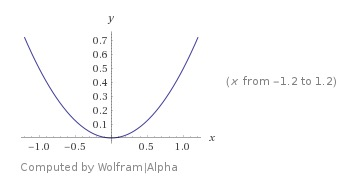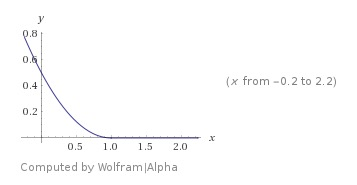Alex Egg,

# Contrastive Loss

Compared to binary x-entropy where my siamese outputs are scalars, in the contrastive loss cases, my siamese outputs are vectors.

Where Y is the binary training label $Y:{0,1}$ where 0 means the pair is identical and 1 not identical. $D_w$ Is the euclidian distance measure of the pair vectors and $m$ is a margin hyper parameter.I the Y=0 case above, meaning the pair is identical, any derivation from $D_w=0$ should be penalized and that’s what the convex $\frac{1}{2}x^2$ cost function indeed does.

In the Y=1 case, where the pairs are not-identical, the loss is inversely proportion to the distance of the vectors. However, in this case it is non-convex.

The base network in this example https://hackernoon.com/facial-similarity-with-siamese-networks-in-pytorch-9642aa9db2f7 actually takes as input two vectors (image embeddings) returns two vectors. You can then run nearest neighboors on these two vectors for a simularity check.

In the example arch, the input to the net is two images, which are then passed through the CNN as a feature extractor. They image embeddings are then passed through a 3 layer MLP which outputs a 5D vector. SO for each pair we get to 5D vectors which we then run Euclidian distance on to determine similarity.